Find Paper, Faster
Example：10.1021/acsami.1c06204 or Chem. Rev., 2007, 107, 2411-2502
BOUNDEDNESS OF MAXIMAL FUNCTIONS ON NONDOUBLING PARABOLIC MANIFOLDS WITH ENDS
Journal of the Australian Mathematical Society  (IF0.8),  Pub Date : 2020-03-11, DOI: 10.1017/s144678872000004x
HONG CHUONG DOAN

Let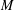$M$ be a nondoubling parabolic manifold with ends. First, this paper investigates the boundedness of the maximal function associated with the heat semigroup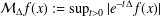${\mathcal{M}}_{\unicode[STIX]{x1D6E5}}f(x):=\sup _{t>0}|e^{-t\unicode[STIX]{x1D6E5}}f(x)|$ where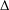$\unicode[STIX]{x1D6E5}$ is the Laplace–Beltrami operator acting on$M$ . Then, by combining the subordination formula with the previous result, we obtain the weak type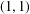$(1,1)$ and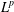$L^{p}$ boundedness of the maximal function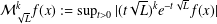${\mathcal{M}}_{\sqrt{L}}^{k}f(x):=\sup _{t>0}|(t\sqrt{L})^{k}e^{-t\sqrt{L}}f(x)|$ on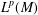$L^{p}(M)$ for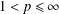$1<p\leq \infty$ where$k$ is a nonnegative integer and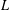$L$ is a nonnegative self-adjoint operator satisfying a suitable heat kernel upper bound. An interesting thing about the results is the lack of both doubling condition of$M$ and the smoothness of the operators’ kernels.